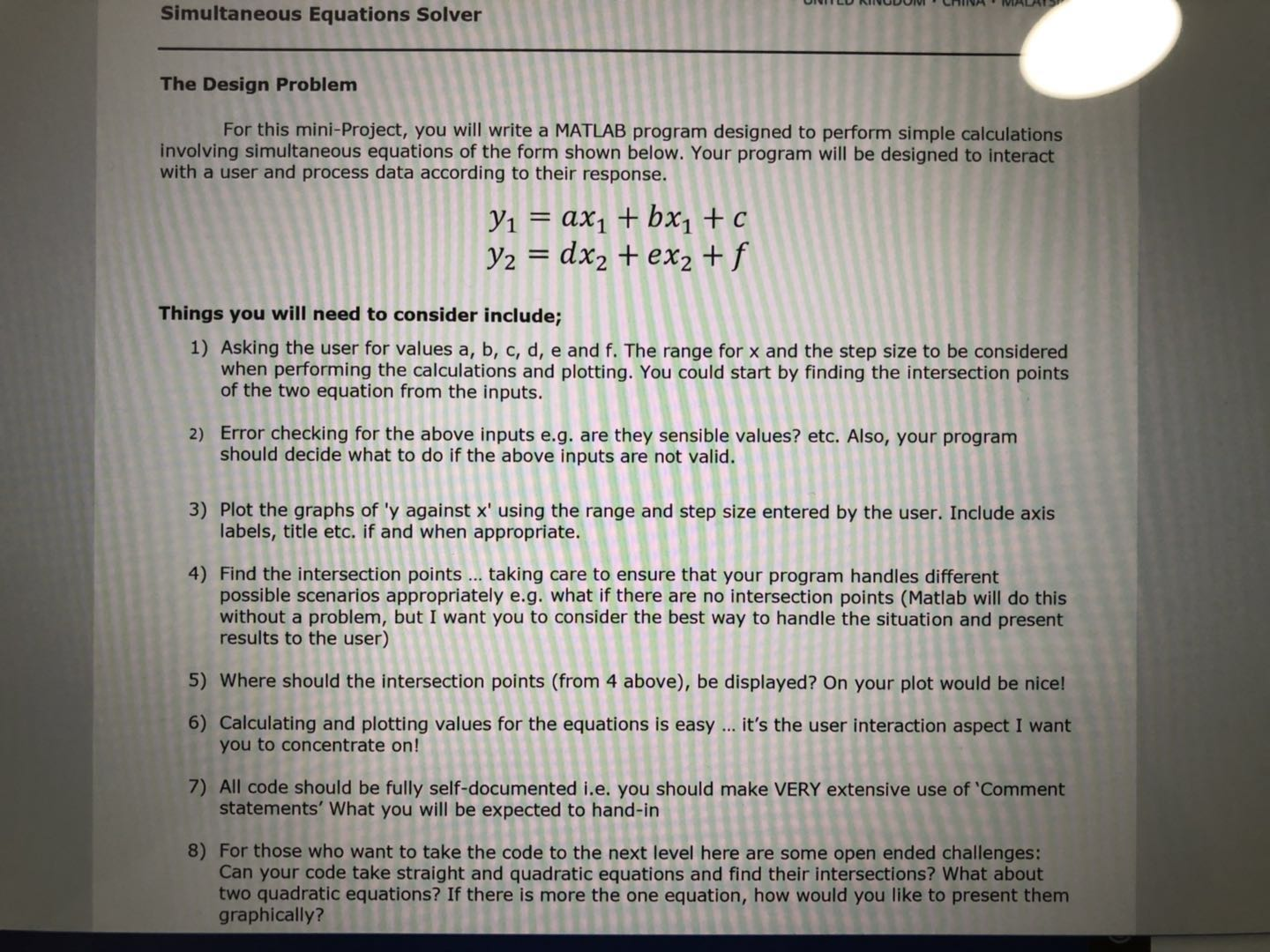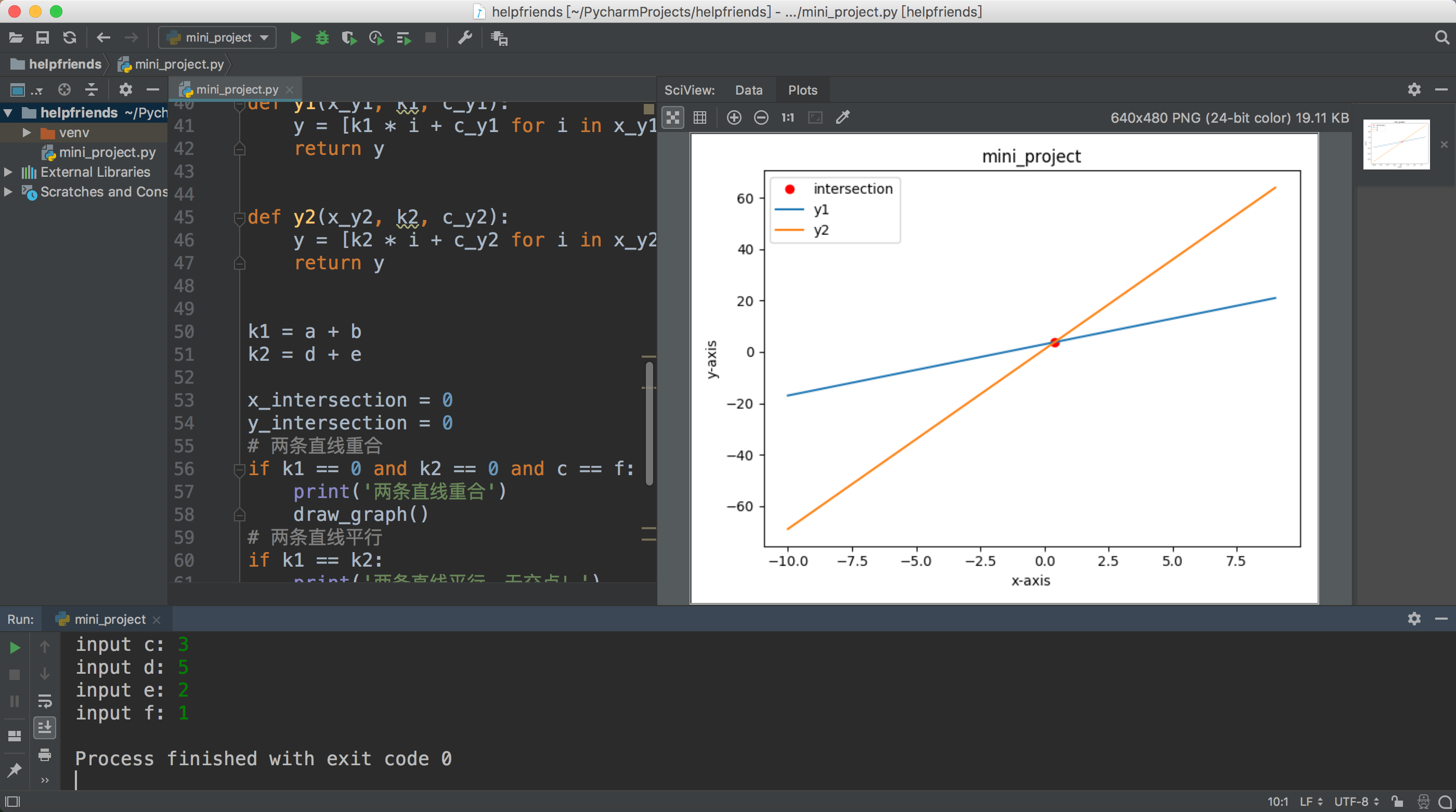• 两直线的交点的计算公式

千次阅读 2020-05-11 10:36:49
bool find_crossPoint(Point p1, Point p2, Point p3, Point p4, Point &crossPoint){ //*************************... // 求二条直线交点公式 // 有如下方程 (x-x1)/(y-y1) = (x2-x1)/(y2-y1) ==> a1*...

bool find_crossPoint(Point p1, Point p2, Point p3, Point p4, Point &crossPoint){
//****************************************************************************************
//  求二条直线的交点的公式
//  有如下方程 (x-x1)/(y-y1) = (x2-x1)/(y2-y1) ==> a1*x+b1*y=c1
//            (x-x3)/(y-y3) = (x4-x3)/(y4-y3) ==> a2*x+b2*y=c2
//  则交点为
//                x= | c1 b1|  / | a1 b1 |      y= | a1 c1| / | a1 b1 |
//                   | c2 b2|  / | a2 b2 |         | a2 c2| / | a2 b2 |
//
//   a1= y2-y1
//   b1= x1-x2
//   c1= x1*y2-x2*y1
//   a2= y4-y3
//   b2= x3-x4
//   c2= x3*y4-x4*y3

float a1 = p2.y - p1.y;
float b1 = p1.x - p2.x;
float c1 = p1.x*p2.y - p2.x*p1.y;
float a2 = p4.y - p3.y;
float b2 = p3.x - p4.x;
float c2 = p3.x*p4.y - p4.x*p3.y;
float det= a1*b2 - a2*b1;

if(det == 0) return false;

crossPoint.x = (c1*b2 - c2*b1)/det;
crossPoint.y = (a1*c2 - a2*c1)/det;

// Now this is cross point of lines
// Do we need the cross Point of segments(need to judge x,y within 4 endpoints)
// 是否要判断线段相交
if((abs(crossPoint.x -(p1.x+p2.x)/2) <= abs(p2.x-p1.x)/2) &&
(abs(crossPoint.y -(p1.y+p2.y)/2) <= abs(p2.y-p1.y)/2) &&
(abs(crossPoint.x -(p3.x+p4.x)/2) <= abs(p4.x-p3.x)/2) &&
(abs(crossPoint.y -(p3.y+p4.y)/2) <= abs(p4.y-p3.y)/2))
{
return true;
}

return false;
}
————————————————
版权声明：本文为CSDN博主「hjk61314」的原创文章，遵循 CC 4.0 BY-SA 版权协议，转载请附上原文出处链接及本声明。
原文链接：https://blog.csdn.net/hjk61314/article/details/82254381

展开全文• 求两条直线方程的交点

千次阅读 2019-02-09 13:20:27
帮一位小伙伴写的作业 题目要求： python3代码实现： &quot;&quot;&quot; y1 = a * x1 + b * x1 + c ... 1. 用户输入a,b,c,d,e,f来得到个方程的交点 2. 对于用户的输入进行错误异常处理 ...

帮一位英国留学的小伙伴写的作业

题目要求：python3代码实现：

"""
y1 = a * x1 + b * x1 + c = (a+b) * x1 + c
y2 = d * x2 + e * x2 + f = (d+e) * x2 + f
## 条件约束：
1. 用户输入a,b,c,d,e,f来得到两个方程的交点
2. 对于用户的输入进行错误异常处理
3. 画坐标系以及两条直线方程
4. 找到交点，确保程序能够对不同的情形进行处理，例如没有交点，即两条直线平行的情况
5. 找到的交点，能够在图上突出的展示出来最好了
6. 关注用户交互方面
7. 归档，并及时交作业
8. 更多挑战，可以尝试考虑求二次方程的交点
"""

import numpy as np
import matplotlib.pyplot as plt

a = int(input('input a: '))
b = int(input('input b: '))
c = int(input('input c: '))
d = int(input('input d: '))
e = int(input('input e: '))
f = int(input('input f: '))

# 创建x坐标
x = np.arange(-10, 10, 1)

def draw_graph():
plt.title('mini_project')
plt.plot(x, y1(x, k1, c), label='y1')
plt.plot(x, y2(x, k2, f), label='y2')
# plt.plot(x_intersection, y_intersection, 'ro', label='intersection')
plt.xlabel('x-axis')
plt.ylabel('y-axis')
plt.legend()
plt.show()

def y1(x_y1, k1, c_y1):
y = [k1 * i + c_y1 for i in x_y1]
return y

def y2(x_y2, k2, c_y2):
y = [k2 * i + c_y2 for i in x_y2]
return y

k1 = a + b
k2 = d + e

x_intersection = 0
y_intersection = 0
# 两条直线重合
if k1 == 0 and k2 == 0 and c == f:
print('两条直线重合')
draw_graph()
# 两条直线平行
if k1 == k2:
print('两条直线平行，无交点！')
# 有交点：
if k1 != k2:
x_intersection = (f - c) / (k1 - k2)
y_intersection = k1 * x_intersection + c
plt.plot(x_intersection, y_intersection, 'ro', label='intersection')
draw_graph()

实现结果：展开全文• 题目：直线与椭圆交点距离公式马上考试了解答：(根号(k^+1))*根号(x1^2+X2^2-2x1x2)再问： 可以说清楚点吗再答： (根号(k^+1))*根号((x1+X2)^2-2x1x2)k为直线的斜率，x1+X2 x1x2用根与系数的关系求即可猜你喜欢：1....

题目：

直线与椭圆交点距离公式

马上考试了

解答：

(根号(k^+1))*根号(x1^2+X2^2-2x1x2)

再问： 可以说清楚点吗

再答： (根号(k^+1))*根号((x1+X2)^2-2x1x2)k为直线的斜率，x1+X2 x1x2用根与系数的关系求即可

猜你喜欢：

1.怎么连起来1.Mark2.learn to play the violin3.take violin lessons.

2.如图在平面直角坐标系中一次函数y=-2分之1x+6的图像分别交x,y轴于点A,B

3.钢琴课在什么时候?用英语怎么说?

4.特百惠的杯子如何

5.求阅读题答案

6.两直线的交点坐标与距离公式

7.直线的交点坐标与距离公式

8.双曲线与直线的交点的距离公式?

9.椭圆X^2/a^2+Y^2/b^2=1(a>b>0)左焦点F,若过点F且倾斜角为45度的直线与椭圆交于AB两点F分向量B

10.M(4,3)是直线被椭圆x²+4y²=36所截得的线段的中点,求直线方程

展开全文• 直线的交点公式

千次阅读 2009-09-26 22:03:00
n条直线互不平行且无三线共点的最多交点数max=1+2+……(n-1)=n(n-1)/2

n 条直线互不平行且无三线共点的最多交点数 max=1+2+ …… (n-1)=n(n-1)/2
展开全文• python 计算两直线交点

万次阅读 2018-05-08 19:17:40
最近在做图像的透射变换，有一...因此，需要通过编写一个函数计算两直线交点。首先，我们定义：直线L1：[x1,y1,x2,y2]，直线L2：[x3,y3,x4,y4]简单解释一下原理：点确定一条直线，而每一条直线都可以表示为：Y=kX+...Python 两直线求交点
• 下面是修正后的/// /// 计算直线交点/// /// L1的点1坐标/// L1的点2坐标/// L2的点1坐标/// L2的点2坐标/// public static PointF GetIntersection(PointF lineFirstStar, PointF lineFirstEnd, PointF ...
• 背景介绍最近在水面无人艇(USV)模拟仿真中，用到了一些点和线的关系求解，本文主要讲述一下点确认直线，点到直线距离，直线交点等问题的解决方法，并给出python程序。部分内容非原创，文中给出链接，需要者...
• 直线的交点坐标与距离公式

千次阅读 2020-12-24 15:25:45
直线交点坐标与距离公式一：直线交点坐标：1、设直线分别为1l：1110AxByC，2l：2220AxByC则1l与2l是否有交点，只需看方程组11122200AxByCAxByC是否有唯一解若方程组有...向量交点坐标公式
• 记录：python 求两直线交点

千次阅读 2020-07-17 17:24:15
本人数学渣渣，公式来自知乎大佬，大佬真是太强了。 https://www.zhihu.com/question/381406535/answer/1095948349 用方程法，避开了算K，真是太强了x2。 def calc_abc_from_line_2d(x0, y0, x1, y1): a = y0 - y1 ...python 求两直线交点
• 遂从最简单的直角坐标系直线交点开始，直线1的方程解析式: 2x-y=0;直线2的方程解析式: 4x-5y=9;记录下思考过程版本一//求直角坐标系中直线2x-y=0　和　4x-5y=9的交点坐标$x = 0;$solution = array();while...java两条直线交点
• 作者：henu_wxj链接：https://blog.nowcoder.net/n/9913fe21a2f64ef080ed41baada7bd9a来源：牛客网这个专题讨论用解析几何的方法解决线段，直线和点的...存在种情况：1.情况一： && 时，直线 的方程为 ...
• JAVA 获取两条直线的交点

千次阅读 2020-07-03 14:47:10
JAVA 获取直线交点 代码如下： public class PointIntersect { public static class Point{ private double x; private double y; public Point(double x,double y){ this.x=x; this.y=y; } public...java
• 我相信哪怕一点光，也能驱散学习中的迷雾，我在这分享一点自己的挫见 思路： 这题最大的难点就是abc三个常数要怎么去构造，这里需要数学...只要能知道一条线段的abc参数，便可以计算条线的交点公式推导： .c++ 开发语言 后端
• 高中数学直线与方程直线交点坐标与距离公式两点间的距离PPT课件.pptx
• 求取两条直线的交点坐标

千次阅读 2018-08-31 12:35:45
Opencv学习笔记—–求取直线交点坐标 #include &amp;amp;lt;opencv2\highgui\highgui.hpp&amp;amp;gt; #include &amp;amp;lt;opencv2\opencv.hpp&amp;amp;gt; using namespace std; using ...
• 用面向对象的思维设计相关类，从而实现直线直线直线与圆、直线与矩形的交点。用面向对象的思维设计相关类，从而实现直线直线直线与圆、直线与矩形的交点。用面向对象的思维设计相关类，从而实现直线直线、...
• 先画一条直线，然后画另一条直线两直线交点回显示出来，VC6.0实现的
• 参数方程下直线交点公式(蓝书257) &理解： 这块不是很懂,但书上写的却是过程略 = = ,在贴吧群里问了q巨,给了一张证明的图,感觉理解了好多.图如下: 转载于:...
• 求空间中直线交点交点坐标的问题完全可以用数学的方法求得结果的表达公式来解决啊 设P1(x1,y1,z1),P2(x2,y2,z2) L2:P3(x3,y3,z3),P4（x4,y4,z4） 记xi-xj=xij,ij是下标 （或xij，yij，zij表示该直线的方向...
• Opencv学习笔记-----求取两条直线的交点坐标

万次阅读 多人点赞 2016-12-15 12:06:07
求取二直线交点(基于OpenCv) 理论补充： 直线是否有交点(或线段齐延长线是否相交)是一个Line-line intersection(Wikipedia)问题 在一个2维平面中有直线(点到点、(点到点，这条直线的交点用行列式表示如下：...opencv 两直线交点
• JAVA求两直线交点和三角形内外心的编程代码一.求两直线交点复制代码 代码如下:class Point {double x;double y;public Point() {this.x = 0;this.y = 0;}}class Line {Point a;Point b;public Line() {this.a = new ...
• void jj(ll x1,ll y1,ll x2,ll y2,ll&A,ll&B,ll&C){ A=y2-y1; B=x1-x2; C=x2*y1-x1*y2; } bool px(ll x1,ll y1,ll x2,ll y2){ return x1*y2==x2*y1;...void getjiao(){//...
• 1、判断斜率相同不，y2-y1/x2-x1,y4-y3/x4-x3,相同则无交点，不相同说明直线交点则执行第二步；2、设y=ax+b,通过斜率可求出a的值，通过坐标可以求出b的值，这样个线段所在直线个方程式就得到了；3、通过个...
• 已知点A（x1，y1）、B（x2，y2）为直线L1上个点，点C（x3，y3）、D（x4，y4）为直线L2上个点，求解直线的方程以及交点坐标，直接贴代码 //定义Point2f结构体 struct Point2f { float x; float y; } // ...
• 两直线交点class Point {double x;double y;public Point() {this.x = 0;this.y = 0;}}class Line {Point a;Point b;public Line() {this.a = new Point();this.b = new Point();}//求直线的交点，斜率相同的话...java直线交点...